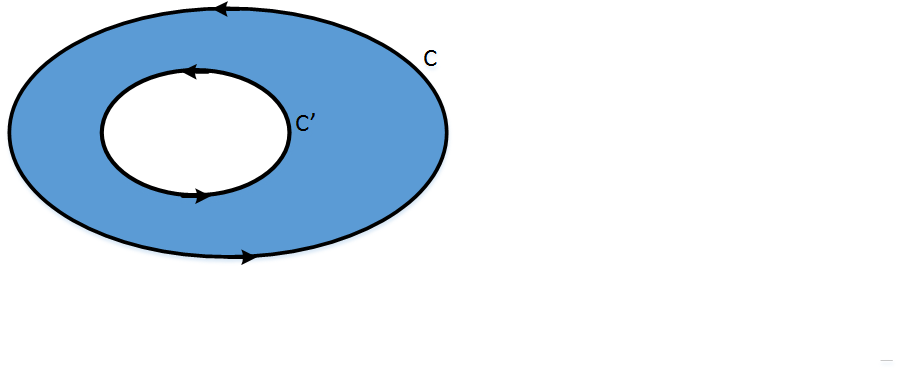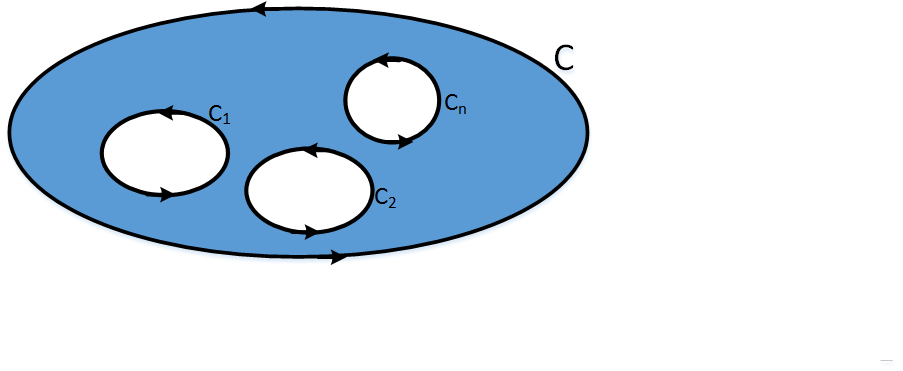# Complex integration

### Basic concepts and principles

One of the most important ways to get involved in complex variable analysis is through complex integration. When we talk about complex integration we refer to the line integral.
Line integral definition begins with γ a differentiable curve such that
$$\begin{matrix}\gamma : [a,b] \mapsto \mathbb{C}\\ \;\;\;\;\; \;\;\;\;\;\;\; x \mapsto \gamma(x) \end{matrix}$$

Now we divide the interval [a, b] in n parts zi such that z0a, and zn=b

For each subinterval we take $E_{i}=f(\zeta_{i})(z_{i}-z_{i-1}), \; i=1,..,n$.

Then we take the partial sums $\sum_{i=1}^{n}E_{i} = \sum_{i=1}^{n}f(\zeta_{i})(z_{i}-z_{i-1})$.Making the limit when n tends to infinity we get the line integral as $$\int_{a}^{b}f(z)dz \;\;, \;\; \int_{C}f(z)dz$$
Both two formulas are analogous

The complex integral over a C curve is defined as

$\int_{C}f(z)dz = \int_{C}(u+iv)(dx+idy)$ $= \int_{C}udx -vdy + i\int_{C}vdx -udy$

A very interesting property of the integral and that is used in most of proofs and arguments is the follwing

$$\left | \int_{a}^{b }f(z)dz \right | \le \int_{a}^{b }\left |f(z) \right |dz$$ Click here to see a proof of this fact.

### Line integral definitionº

Given f, a complex variable function and γ a piecewise differentiable curve. We define the line integral of f over γ as: $$\int_{\gamma}f(z)dz = \int_{a}^{b}f(\gamma(t))\gamma'(t)dt$$

### Extended theory

The most important therorem called Cauchy's Theorem which states that the integral over a closed and simple curve is zero on simply connected domains. Cauchy gave a first demonstration supposing that the function f has a continuous first derivative, later Eduard Gousart discovered that in fact, this hypothesis was redundant, for this reason Cauchy's theorem is sometimes called Cauchy-Gousart's Theorem. This will be the version that we will see here.

In the following theorems, C is a closed and simple curve and contained in a simply connected open R region (this is a domain).

### Theorem of Cauchy - Gousart

Given f a, holomorphic function over R, then $$\int_{C}f(z)dz = 0$$

Click here to see a proof of Cauchy's theorem.

### Green's Theorem in the plane

Let P and Q be continuous functions and with continuous partial derivatives in R and on their boundary C. Then

$\int_{C} P dx+ Q dy$ $= \int\int_{R}[\frac{\partial Q}{\partial x}- \frac{\partial P}{\partial y}]dx dy$

It is relatively simple to put Green's theorem in complex form:

### Green's theorem in complex form

Given F, with continuous partial derivatives in R and on their boundary C. Then

$\int_{C}F(z, \bar{z})dz$ $= 2i\int\int_{R}\frac{\partial F}{\partial \bar{z}}dA$

Click here to see a proof
The following Theorem is sometimes called reciprocal of the Cauchy's theorem

### Morera's Theorem

Given f, a complez variable function, lets suposse that it verifies $$\int_{C}f(z)dz = 0$$ then, f is Holomorphic over R.

### Consequences of the Cauchy's theorem

The following theorems are consequences of Cauchy's theorem

### Theorem 1

If a and b are two points of R then the integral $$\int_{a}^{b} f(z) dz$$ It is independent of the path followed between a and b.
The proof of this theorem is simple, it is enough to observe if C is any path between a and b and C' is another different path, then for Cauchy's theorem, the total integral between C and C' is zero, as the path C 'does not matter, both line integrals would be same.

### Theorem 2

Lets a and b two points of R and F'(z) = f(z) then $$\int_{a}^{b} f(z) dz = F(b) - F(a)$$
Reciprocally, if a and z are points of R and it is fulfilled
$$F(z) = \int_{a}^{z} f(z) dz$$
then, F is Holomorphic in R and F'(z) = f(z)

The following theorem is very important, it talk about that the value of an integral over a closed and simple curve that surrounds a singularity does not depend on the curve

### Theorem 3

Given f a function Holomorphic in a region bounded by two closed and simple curves C and C' $$\int_{C} f(z) dz = \int_{C'} f(z) dz$$ Where C and C' are traversed positively oriented, that is, counterclockwise.

The following theorem is a generalization of the previous one in a region with n singularities instead two.Figura 1: Region closed between curves C and C'

### Teorema 4

Given f a function Holomorphic in a region bounded by nclosed and simple curves $C_{1}, C_{2}, ..., C_{n}$. Which are enclosed by another major curve C. Then.

$\int_{C} f(z) dz = \int_{C_{1}} f(z) dz + \int_{C_{2}} f(z) dz +$ $... + \int_{C_{n}} f(z) dz$

Where the curves curves $C_{1}, C_{2}, ..., C_{n}$ are traversed positively oriented, that is, counterclockwise.Figura 2: Region encerrada entre las curvas C y $C_{1}, C_{2}, ..., C_{n}$

## Was useful? want add anything?

Post here

### kamaljitsingh gill:

2019-10-21 14:33:23
the theorem together make things clear for understanding .The content is explained nicely

### Admin:

2019-08-21 12:33:33
Thanks, kamaljitsingh gill.

Nice to be useful.

Post here